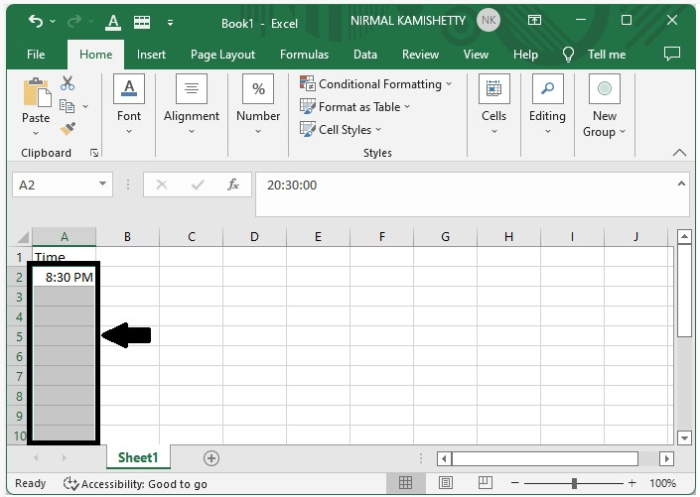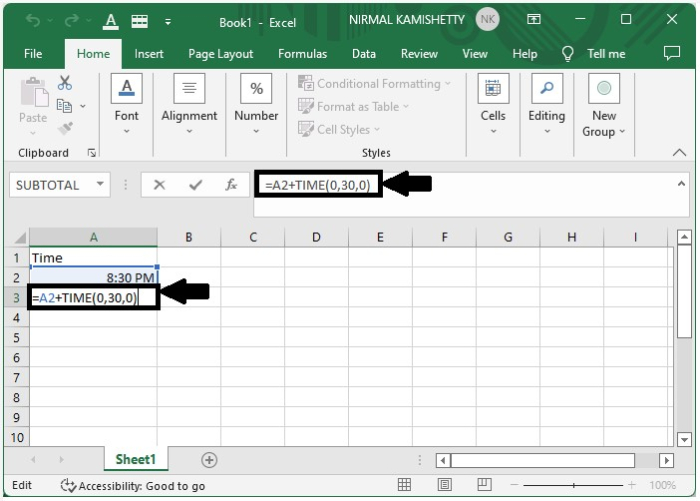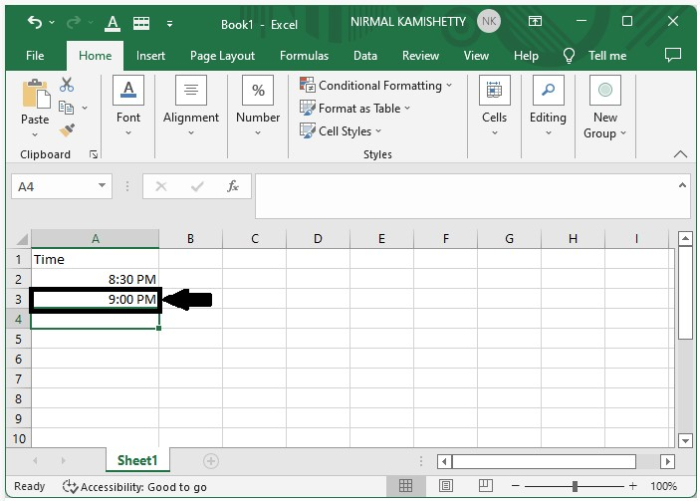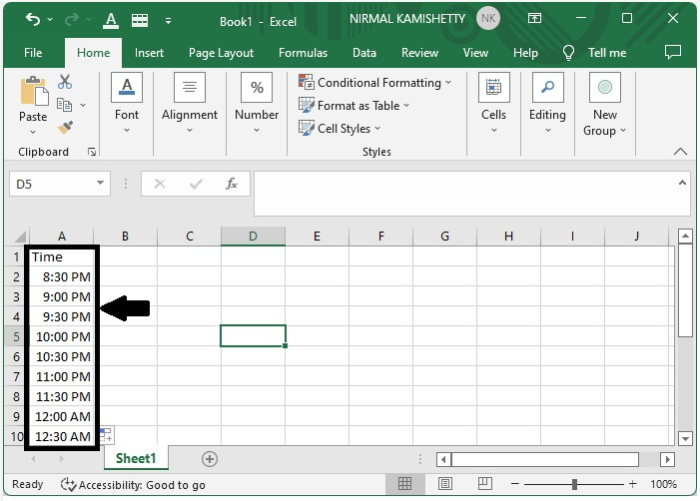# How to add time with hours/minutes/seconds increments in Excel?

Maintaining the value of a particular item at every instance of time is a very crucial process if the prices of the item change very frequently. If we want to note the value of an item in particular intervals, then we need to create a list that contains the time with hours/minutes/seconds increment. For example, such a list is quite useful in case of stock prices because they change rapidly. Although we can’t note every value, we can note the value for all particular intervals, like for every 15 minutes, to analyse its change of price.

## How to Add Time with Hours/Minutes/Seconds Increments?

Let us take a simple example to demonstrate how you can create a list of time with equal intervals.

### Step 1

Open an empty Excel sheet and type the first timestamp that is recorded. Next, select the cells under the first cell based on the number of timestamps that you want to show.### Step 2

In the formula box, enter the formula =A2+TIME (0,30,0) to get the list where time increment is 30 minutes.In our example, we have used the following formula −

=A2+TIME (0,30,0)

Here, "A2" is the address of the first entered timestamp. The first "0" is the change in hours, "30" is the change in minutes, and the second "0" is the change is seconds.

Press the "Enter" button to get the first result, as shown in the following screenshot −### Step 3

And finally, we can get all the other results by pressing "ALT+ENTER" and it is our final result.## Conclusion

This is how you can create a list of equal time intervals in Excel. Not only in minutes, we can create a list for seconds and hour intervals by making small changes in the given formula. We can customize the formula based on our requirements.

Updated on: 23-Dec-2022

12K+ Views